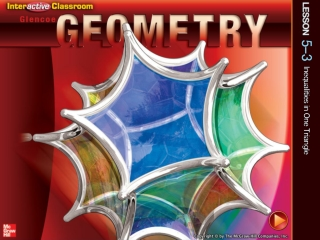DownloadDownload PresentationSplash Screen

# Splash Screen

Télécharger la présentation## Splash Screen

- - - - - - - - - - - - - - - - - - - - - - - - - - - E N D - - - - - - - - - - - - - - - - - - - - - - - - - - -
##### Presentation Transcript

1. Splash Screen

2. Five-Minute Check (over Lesson 5–2) CCSS Then/Now Key Concept: Definition of Inequality Key Concept: Properties of Inequality for Real Numbers Theorem 5.8: Exterior Angle Inequality Example 1: Use the Exterior Angle Inequality Theorem Theorems: Angle-Side Relationships in Triangles Example 2: Order Triangle Angle Measures Example 3: Order Triangle Side Lengths Example 4: Real-World Example: Angle-Side Relationships Lesson Menu

3. Find the coordinates of the centroid of the triangle with vertices D(–2, 9), E(3, 6), and F(–7, 0). A. (–4, 5) B. (–3, 4) C. (–2, 5) D. (–1, 4) 5-Minute Check 1

4. A. B. C.(2, 3) D. Find the coordinates of the orthocenter of the triangle with vertices F(–1, 5), G(4, 4), and H(1, 1). 5-Minute Check 2

5. ___ ___ In ΔRST, RU is an altitude and SV is a median.Find y if mRUS = 7y + 27. A. 5 B. 7 C. 9 D. 11 5-Minute Check 3

6. ___ ___ In ΔRST, RU is an altitude and SV is a median. Find RV if RV =6a + 3 and RT =10a + 14. A. 3 B. 4 C. 21 D. 27 5-Minute Check 4

7. Which of the following points is the center of gravity of a triangle? A. centroid B. circumcenter C. incenter D. orthocenter 5-Minute Check 5

8. Content Standards G.CO.10 Prove theorems about triangles. Mathematical Practices 1 Make sense of problems and persevere in solving them. 3 Construct viable arguments and critique the reasoning of others. CCSS

9. You found the relationship between the angle measures of a triangle. • Recognize and apply properties of inequalities to the measures of the angles of a triangle. • Recognize and apply properties of inequalities to the relationships between the angles and sides of a triangle. Then/Now

10. Concept

11. Concept

12. Concept

13. Use the Exterior Angle Inequality Theorem By the Exterior Angle Inequality Theorem, m14 > m4 and m14 > m11. In addition, m14 > m2 and m14 > m4 + m3, so m14 > m4 and m14 > m3. Since 11 and 9 are vertical angles, they have equal measure, so m14 > m9. m9 > m6 and m9 > m7, so m14 > m6 and m14 > m7. Example 1

14. Use the Exterior Angle Inequality Theorem By the Exterior Angle Inequality Theorem, m10 > m5 and m16 > m10, so m16 > m5. Since 10 and 12 are vertical angles, m12 > m5. m15 > m12, so m15 > m5. In addition, m17 > m5 + m6, so m17 > m5. Example 1

15. A. B. C. D. Example 1

16. A. B. C. D. Example 1

17. Concept

18. The sides from the shortest to longest are AB, BC, and AC. The angles opposite these sides are C,A, and B, respectively. So, according to the Angle-Side Relationship, the angles from smallest to largest are C, A, B. Order Triangle Angle Measures List the angles of ΔABC in order from smallest to largest. Answer:C, A, B Example 2

19. List the angles of ΔTVX in order from smallest to largest. A. X, T, V B. X, V, T C. V, T, X D. T, V, X Example 2

20. The angles from smallest to largest are B,C, and A. The sides opposite these angles are AC, AB, and BC, respectively. So, the sides from shortest to longest are AC, AB, BC. Answer:AC, AB, BC Order Triangle Side Lengths List the sides of ΔABC in order from shortest to longest. Example 3

21. A.RS, RT, ST B.RT, RS, ST C.ST, RS, RT D.RS, ST, RT List the sides of ΔRST in order from shortest to longest. Example 3

22. Angle-Side Relationships HAIR ACCESSORIES Ebony is following directions for folding a handkerchief to make a bandana for her hair. After she folds the handkerchief in half, the directions tell her to tie the two smaller angles of the triangle under her hair. If she folds the handkerchief with the dimensions shown, which two ends should she tie? Example 4

23. Angle-Side Relationships Theorem 5.10 states that if one side of a triangle is longer than another side, then the angle opposite the longer side has a greater measure than the angle opposite the shorter side. Since Xis opposite the longest side, it has the greatest measure. Answer: So, Ebony should tie the ends marked Y and Z. Example 4

24. KITE ASSEMBLY Tanya is following directions for making a kite. She has two congruent triangular pieces of fabric that need to be sewn together along their longest side. The directions say to begin sewing the two pieces of fabric together at their smallest angles. At which two angles should she begin sewing? A. A and D B. B and F C. C and E D. A and B Example 4

25. End of the Lesson